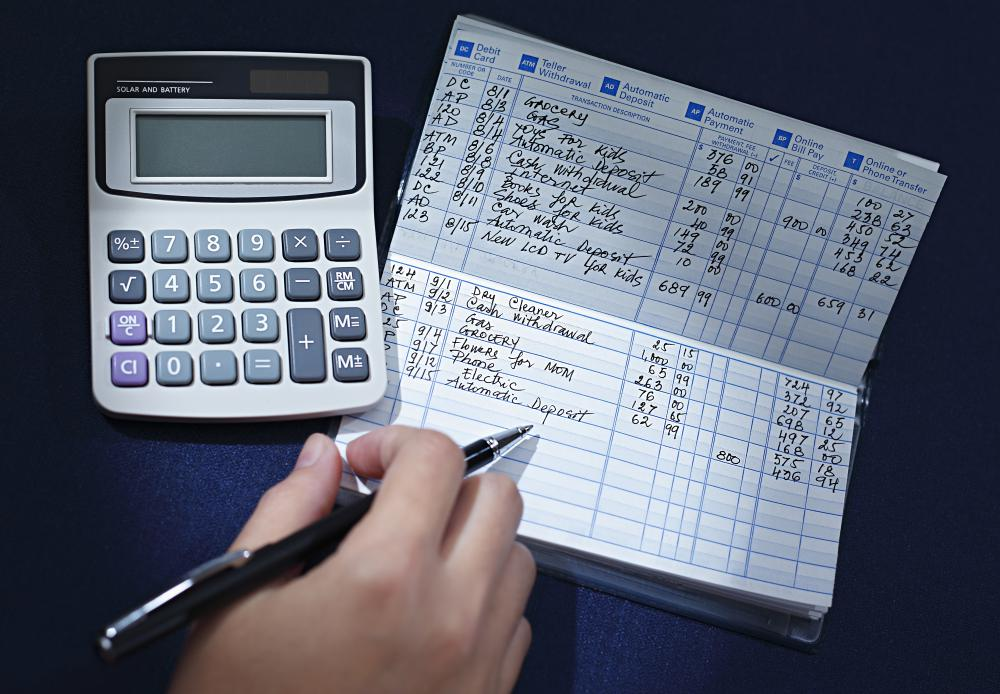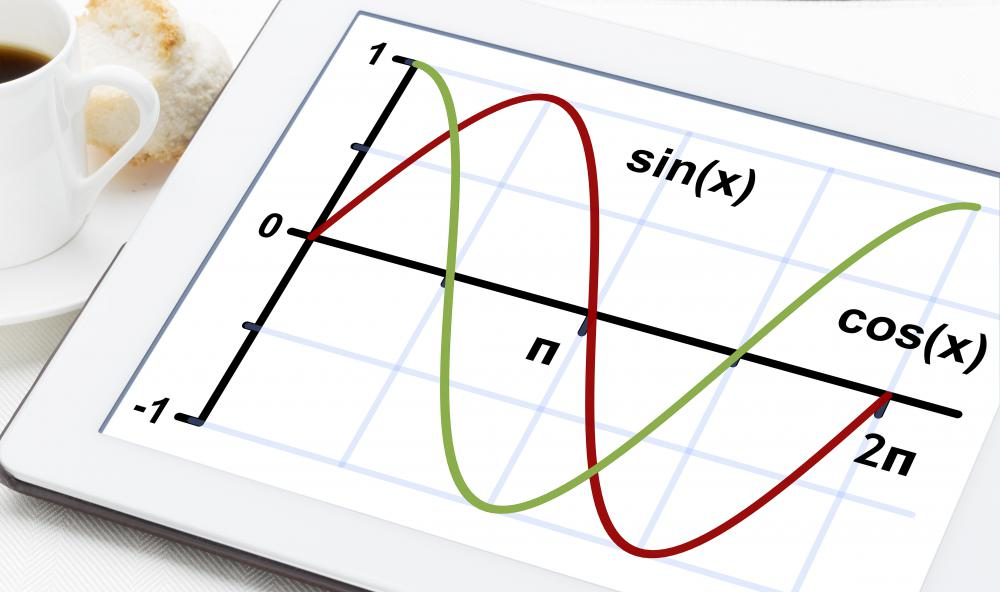# What is a Handheld Calculator? (with pictures)

Matthew F.
Matthew F.A handheld calculator might include functions to help solve algebra problems.

A handheld calculator is a small device used for solving mathematical equations. Its range of calculations can be simple or complex, depending on the capabilities of the calculator. These calculators have come to be important assets for high school and college mathematics students. Since its introduction to mainstream consumers, the handheld calculator has become a valuable tool for many household mathematical problems as well, such as budgeting and balancing checkbooks.Many people use a handheld calculator to help them balance a checkbook.

Calculators, which were first introduced as mechanical machines in the early 1900s, were often large, impractical, and expensive. Electronic calculators first emerged in the 1950s, but were still often the size of a typewriter. In 1967, the first handheld calculator was developed by Texas Instruments, the company that is still among the leaders in handheld calculator production, innovation, and technology. This first calculator could do the four basic functions -- addition, subtraction, multiplication, and division -- and produced a paper output.Many handheld calculators are equipped with tools for trigonometric functions.

As the handheld calculator began to be integrated into fewer and fewer chips and internal functions, calculators became smaller, cheaper and more available to the general public. In 1970, the first portable calculators appeared with rechargeable batteries. Technology continued to develop the handheld calculator through the 1970s and 1980s to the model that has been common since the 1990s.

A handheld calculator can come in different shapes and sizes, with different capabilities. They can be made to sit up on a desk, or can be made with larger numbers for the hard-of-seeing or less nimble. They always feature the basic 0-9 digits, with keys for decimals, addition, subtraction, division, multiplication, percentages, memory, negatives, and a cancel or clear button. They utilize a small liquid crystal display (LCD), with an internal circuit board and integrated chips for memory and calculation.

Many handheld calculators, though, are also equipped with tools for trigonometry, statistics, or algebra. These are common in higher-level calculus or trigonometry classes, and can calculate exponents, logarithms, scientific notations, and graphing. Known as scientific calculators, this type of handheld calculator works much like more simple calculators. They offer the ability to input data through numbered buttons; the ability to decide which function to perform, such as division or multiplication; and the ability to solve the equation in fractions of a second.

Different from the computing and memory-based functions that a computer is capable of, the handheld calculator is capable of calculating general output from different inputs. However, as technology continues to evolve, the handheld calculator has been integrated into computers and cell phones in the 2000s. A simple calculator can now be found within most cellular devices, with at least addition/subtraction/multiplication/division functions. They are becoming more and more capable of computing through functions that require programming as well.

## You might also Like## Discuss this Article

Forgot password?
###### Register:
•A handheld calculator might include functions to help solve algebra problems.
•Many people use a handheld calculator to help them balance a checkbook.
•Many handheld calculators are equipped with tools for trigonometric functions.